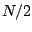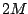Next: Transition splicing Up: Predicting and controlling foldover Previous: Over-sampling   Contents   Index

Sneaky triangle waves

For low fundamental frequencies, over-sampling is an easy way to get adequate foldover protection. If we wish to allow higher frequencies, we will need a more sophisticated approach. One possibility is to replace discontinuities by ramps, or in other words, to replace component sawtooth waves by triangle waves, as treated in Section 10.3.4, with values ofsmall enough that the result sounds like a sawtooth wave, but large enough to control foldover.

Returning to Figure 10.8, suppose for example we imitate a sawtooth wave with a triangle wave withequal to two samples, so that the first notch falls on the Nyquist frequency. Partials above the first notch (the 17th partial in the figure) will fold over; the worst of them is about 40 dB below the fundamental. On the other hand, the partial strengths start dropping faster than those of a true sawtooth wave at about half the Nyquist frequency. This is acceptable in some, but not all, situations.

The triangle wave strategy can be combined with over-sampling to improve the situation further. Again in the context of Figure 10.8, suppose we over-sample by a factor of 4, and set the first notch at the original sample rate. The partials up to the Nyquist frequency (partial 8, at the fundamental frequency shown in the figure) follow those of the true sawtooth wave fairly well. Foldover sets in only at partial number 48, and is 52 dB below the fundamental. This overall behavior holds for any fundamental frequency up to about one quarter the sample rate (after whichexceeds). Setting the notch frequency to the original sample rate is equivalent to setting the segment of lengthto one sample (at the original sample rate).Next: Transition splicing Up: Predicting and controlling foldover Previous: Over-sampling   Contents   Index
Miller Puckette 2006-12-30